# Counterexample, Fixed-Network Connection Model

Tom Kelliher, CS 315

Feb. 8, 1999

### Assignment

Homework due 2/17: Problems 1.2, 1.3, 1.5, 1.7, 1.15.

### From Last Time

Lower bound techniques, counterexample.

### Outline

1. The counterexample.

2. Properties of the fixed-network connection model.

# A Counterexample

Consider sorting within a complete binary tree: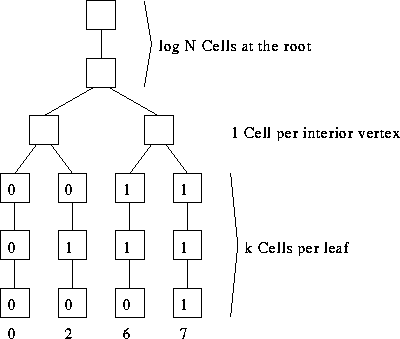1. Assumption: All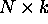bits appear simultaneously.

2. I/O bandwidth? Diameter? Bisection width?

3. Lower bound for sorting on a binary tree?

4. Almost right. Holds for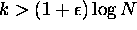.

5. So, how do we beat the lower bound? By counting.

## Example: k=1

1. Problem of unary to binary conversion.

2. ``Easy'' if interior cells are word processors.

3. Each interior processor need only be a serial adder and we do bit-wise serial addition with numbers transmitted lsb-first.

Example: Bit-wise addition of 0110 and 0011.

4. Example: counting the 1's when N = 8.

5. Setting the leaf bits:
1. Assume we have m 1's.

2. Set the rightmost m leaves. Clear the leftmost N - m leaves.

3. Observation: right 0 leaf is numbered N - 1 - m.

This is the bit-wise complement of m.

4. Use N - 1 - m to find a path to that leave, clearing leaves to the left, setting leaves to the right, and clearing that leave.

## Generalizing to Larger k

1. Sorting can be done in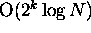. What does this tell us?

2. Don't forget: You're counting, not sorting.

# Properties of the Fixed-Connection Network Model

1. Processor properties:
1. Local control.

2. Computation is a function of local storage and local inputs.

3. Variations. Processor might know such things as its address, topology/size of the network, time.

Storage is usually constant, but may vary with size of network.

4. A bit processor may perform a constant number of bit operations per step. Similarly for a word processor.

5. Limits on word size usually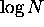.

6. Packets: indivisible communication data stamped with a key.

2. Interconnection properties:
1. Connections may not vary with time.

2. A constant amount of communication (bits, words, packets, as appropriate) can occur across a connection per step.

3. Bounded-degree network. Sometimes degree will be O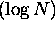.

Size is polynomial in N.

3. I/O protocol properties:
1. Each input is provided only once.

2. I/O is when and where oblivious: the time and location, for each piece of data, of I/O must be specified in advance.

Without this restriction, we could sort in constant time.

4. Comment: This model describes systolic VLSI very well. It doesn't describe general VLSI well since we don't have ``long'' wires. But, how does one model the communication properties of a long wire?

Thomas P. Kelliher
Mon Feb 8 07:57:37 EST 1999
Tom Kelliher